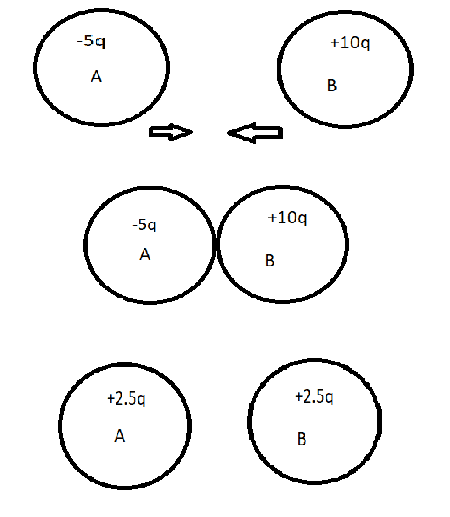# Electrical conductivity has an effect while charging by conduction?

kololo
Homework Statement:
Does electrical conductivity affects only the time taken for two conductors to obtain their resultant charge?
Relevant Equations:
-
I understand that electrical conductivity is a measure of how easily charges are able to move in an object.
During charging by conduction (for conductors), it seems that we simply have to add the charges of both object together and divide by two to obtain the resultant charge of either object. Does electrical conductivity then affects only the time taken for the two conductors to obtain their resultant charge?

•Delta2

Staff Emeritus
2022 Award
To charge an object you need to have an electric current into and through that object, correct? How does the resistance (or conductivity) affect an electric current?

•Delta2, kololo and scottdave
Gold Member
"resultant charge", to me, means steady state, or that nothing is changing any more, the system is stable. What can you conclude from that assumption about your problem?

•kololo
kololo
To charge an object you need to have an electric current into and through that object, correct? How does the resistance (or conductivity) affect an electric current?Do you mean to say that when the particles are in contact with each other and when electrons move from A to B, there is electron current from A to B?
Since the current is voltage divided by resistance, the higher the conductivity, the less the resistance, and thus the higher the current. And since current is the rate of flow of charge, a higher current will mean that it will take a shorter time for A and B to obtain 2.5q of charge? Please correct me if I'm wrong.

•Delta2
Staff Emeritus
2022 Award
Do you mean to say that when the particles are in contact with each other and when electrons move from A to B, there is electron current from A to B?
Absolutely. Electrons are electric charges after all, and current is defined as the movement of electric charges.

Since the current is voltage divided by resistance, the higher the conductivity, the less the resistance, and thus the higher the current. And since current is the rate of flow of charge, a higher current will mean that it will take a shorter time for A and B to obtain 2.5q of charge? Please correct me if I'm wrong.
That looks correct to me.

•Delta2 and kololo
kololo
Absolutely. Electrons are electric charges after all, and current is defined as the movement of electric charges.

That looks correct to me.
Alright, I understand now. Thank you!

•Delta2
Homework Helper
Gold Member
BTW I don't think its an easy problem to find a formula for the current that flows when we bring two conductors of different potential in contact , but I agree that the conductivity of the conductors will have a proportional effect to this current. Or the resistivity will have an inversely proportional effect. The reason for this is I think Ohm's law in its differential form $$\mathbf{J}=\sigma\mathbf{E}$$ where it gives us clearly that the current density ##\mathbf{J}## is proportional to the conductivity ##\sigma##.

For a simplified version of this problem, check the following thread

PS. Sorry the above link is for two spherical conductors connected by a coil of inductance L but the solution path would be similar if we assume they are connected by a conductor of ohmic resistance R.

Last edited:
•kololo
kololo
BTW I don't think its an easy problem to find a formula for the current that flows when we bring two conductors of different potential in contact , but I agree that the conductivity of the conductors will have a proportional effect to this current. Or the resistivity will have an inversely proportional effect. The reason for this is I think Ohm's law in its differential form $$\mathbf{J}=\sigma\mathbf{E}$$ where it gives us clearly that the current density ##\mathbf{J}## is proportional to the conductivity ##\sigma##.

For a simplified version of this problem, check the following thread
•